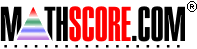Math Practice Online > free > lessons > Florida > 6th grade > Unit Cost

## Unit Cost

Word problems related to the unit cost of things. You may need pencil and paper.

 Sample Problems for Unit Cost Lesson for Unit Cost

### This topic aligns to the following state standards

Grade 5: Num 1. Solves real-world problems involving addition, subtraction, multiplication, and division of whole numbers, and addition, subtraction, and multiplication of decimals, fractions, and mixed numbers using an appropriate method (for example, mental math, pencil and paper, calculator).
Grade 4: Meas 1. Selects an appropriate measurement unit for labeling the solution to real-world problems.
Grade 5: Meas 1. Selects an appropriate measurement unit for labeling the solution to real-world problems.
Grade 6: Num 1. Knows the appropriate operations to solve real-world problems involving whole numbers, decimals, and fractions.
Grade 6: Num 2. Solves real-world problems involving whole numbers, fractions, decimals, and common percents using one or two-step problems.
Grade 7: Num 1. Knows the appropriate operation to solve real-world problems involving fractions, decimals, and integers.
Grade 6: Num 1. Solves one- or two-step real-world problems involving whole numbers and decimals using appropriate methods of computation (for example, mental computation, paper and pencil, and calculator).

Copyright Accurate Learning Systems Corporation 2008.